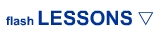ADVANCED/GAME Lesson 1: Control Movieclips Lesson 2: Flip Symbol Lesson 3: Inside Movieclip Lesson 4: Dynamic Text Lesson 5: Random Clip Lesson 6: Actionscript Music Lesson 7: Symbol Timer Lesson 8: Rotate Movieclip Lesson 9: Disable Right-click Lesson 10: Frame Names Lesson 11: Side Scroller Lesson 12: Increasing Velocity Lesson 13: Visibility Lesson 14: What's 'this' Lesson 15: Collectable Items Lesson 16: Specified Action Lesson 17: Button Tap Code Lesson 18: Random in Detail Lesson 19: Health Bar Lesson 20: Follow Movieclips MORE GAMES Lesson 1: Movement Lesson 2: Wall Collision Lesson 3: Shoot Lesson 4: Shot Collision Lesson 5: Score ANIMATION IN FLASH Lesson 1: Character Lesson 2: Eye Movement Lesson 3: Arm Movement Lesson 4: Walking Lesson 5: Background Lesson 6: Loop Background ROB's CORNER Introduction Lesson 1: Orbital Dots 1 Lesson 2: Orbital Dots 2 Lesson 3: Spinning 1 Lesson 4: Spinning 2 Lesson 5: Easing Spaceship Lesson 6: Sprite 3d Lesson 7: Bouncy Ball Lesson 8: Bouncy Ball 2 2 Lesson 9: Simple Collision Lesson 10: Crazy Tiles ****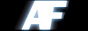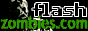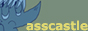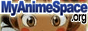Link Us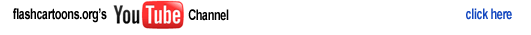home > learn flash > actionscript 3.0 lesson 4

Actionscript 3.0 Lesson Four: If Statements

 1.)If statements are a way of telling the program what to do on certain conditions. You can tell the program to do something while it equals something, or is less than or greater than, while it is greater than or equal to something, while it is less than or equal to something, or not equal. Here is a few examples in this example code. You can test this by placing it inide a actionscript3.0 document's frame. var myString:String = "text"; var myInt:int = 100; if(myString=="text") { trace("the variable is the same as the String"); } if(myInt != 100){ trace("the variable is not equal to the int"); } if(myInt <= 25){ trace("the variable is greater than or equal to 25"); } --------- --------------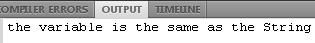--------- -------------- 2.) The Breakdown. In this example the trace example will be executed, as well as the 2nd if statement, and the 3rd example will not be executed, because the variable myInt is not less than or equal to 25. You can also do shortcuts to typing your actionscript file. such as telling the program straight up if you want the variable to be exectuted if it is true. Such as this example I will provide. And you can also add statements when the program also has another option of being with an else statement. var myBoolean:Boolean = true; if(myBoolean){ trace("my Boolean equals true"); } else if(!myBoolean){ trace("my Boolean equals false;"); }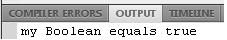3.) In an if statement if you want to say the variable may be false you place a explanation point before the called variable. In this example the first if statement was executed and the second if statement was not called because the called variable did equal true; -jordan